#### Interpretation in terms of I-spaces

An alternative formulation will now be given to help understand the connection to I-spaces of a set of environments. The state space, as defined previously, could instead be defined as a configuration space,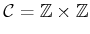. Let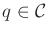denote a configuration. Suppose that each possible environment corresponds to one way to assign costs to all of the edges in a configuration transition graph. The set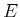of all possible environments for this problem seems to be all possible ways to assign costs,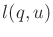. The state space can now be defined as, and for each state,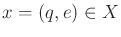, the configuration and complete set of costs are specified. Initially, it is guessed that the robot is in some particular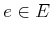. If a cost mismatch is discovered, this means that a different environment model is now assumed because a transition cost is different from what was expected. The costs should actually be written as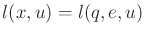, which indicates the dependency of the costs on the particular environment is assumed.

A nondeterministic I-state corresponds to a set of possible cost assignments, along with their corresponding configurations. Since the method requires assigning costs that have not yet been observed, it takes a guess and assumes that one particular environment in the nondeterministic I-state is the correct one. As cost mismatches are discovered, it is realized that the previous guess lies outside of the updated nondeterministic I-state. Therefore, the guess is changed to incorporate the new cost information. As this process evolves, the nondeterministic I-state continues to shrink. Note, however, that in the end, the robot may solve the problem while being incorrect about the precise. Some tiles are never visited, and their true costs are therefore unknown. A default assumption about their costs was made to solve the problem; however, the truecan only be known if all tiles are visited. It is only true that the final assumed default values lie within the final nondeterministic I-state.

Steven M LaValle 2020-08-14